# Profit and Loss Questions with Solutions

Profit and Loss, all in all, make approximate 1 or 2 questions that are standalone as in word problem. These questions can be easily solved using one of the most popular topics in quantitative aptitude which is PERCENTAGE.
I hope you have had a handful of problems based on Percentage which will make this topic easier and help you secure all the marks allotted to Profit and Loss problems.
Before jumping to the hard-core Profit-Loss problems, let us first discuss the basic terminologies related to the topic.

• Cost Price – This is the rate at which the commodity is bought.
• Selling Price – This is the rate at which the commodity is sold.
• Profit – When the Cost Price of the commodity is less than the Selling Price.
• Profit = Selling Price – Cost Price
• Loss – When the Cost Price of the commodity is greater than the Selling Price.
• Loss = Cost Price – Selling Price

In case of competitive exams, one another term is used which is#### Points to Remember

• If there is a PROFIT of x%, the calculating figures would be 100 and (100 + x).
• If there is a LOSS of x%, the calculating figures would be 100 and (100 - x).
• Calculating figures be Cost Price and Selling Price respectively.

Without any delay, let us jump to the questions starting from the basic ones and then proceeding to the higher level.

### Question 1:

A man buys a toy for Rs. 25 and sells it for Rs. 30. Find his gain/loss percentage.
Solution: As we can see, the Cost Price is smaller than Selling Price; therefore the person must have had a profit on the total transaction. Therefore using the above mentioned formula### Question 2:

When an article is old at Rs. 910000, then the profit percentage amounts to 30%. Determine the Cost Price.
Solution: When profit percentage is x%, in this case 30%,
Cost Price = 100
Selling Price = 100 + x = 100 + 30 = 130
The value of 130% is 910000, to find the value of Cost Price i.e. 100% the equation must be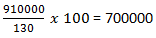### Few Practice Problems:

1. Question: Calculate the Cost Price when the Selling price of the article is Rs. 42500 and loss percentage on selling the article amounts to 15%.
2. Question: When the article is bought at Rs. 8000 and sold at Rs. 9500, calculate the gain percentage.
3. Question : A man got a radio for Rs. 800 and spent Rs. 400 on its repair. He sold it for Rs. 1500. Find his gain percentage.

Before moving forward, let us solve some more questions based on the similar logic.

### Question 3

A man bought a cycle for Rs. 250. For how much should he sell it so as to gain 10%?
Solution: As we have discussed above, the formula shows
Profit percentage = x% = 10%
Cost Price = 100
Selling Price = 100 + x = 100 + 10 = 110
Cost Price is provided to us as Rs. 250 and according to our calculation amounts to 100%. So, the equation becomes### Few Practice problems:

1. Question: A man bought a bicycle for Rs. 560. For how much should he sell it so as to lose 10%?
[HINT: The formulae for Profit and Loss differ a little bit. Check them before starting the calculation.]
2. Question: By selling an article for Rs. 390 a shopkeeper gains 20%, Find the Cost Price?
Another very common question asked in the exams also called “Goods passing through successive hands”. Let us explore the type.

### Question 4

Ram buys a watch for Rs. 500 and sells it to Shyam at 10% loss. Shyam then sells it to Ravi at 20% profit and Ravi sells it to Rakesh at 10% profit. How much did Rakesh pay for the watch?
Solution: Rather than using the amount for calculation, use percentages to make it easier.
Let us consider that Ram spends 100 to buy the watch.
➡Ram buys watch at 100 and sells it to Shyam at 10% loss. 10% of 100 is 10. Therefore,
➡ Cost Price for Ram = 100
➡ Selling Price for Ram = 100 – (10% of 100 = 10) = 100 - 10 = 90
➡ Cost Price for Shyam = 90
➡ Selling Price for Shyam = 90 + (20% of 90 = 18) = 90 + 18 = 108
➡ Cost Price for Ravi = 108
➡ Selling Price for Ravi = 108 + (10% of 108 = 10.8) = 108 + 10.8 = 118.8
➡ Cost Price for Rakesh = 118.8
➡ The initial amount was Rs. 500 and the percentage we considered 100. So for final calculation, the equation becomes,➡ So, the amount Rakesh spent to buy the watch is Rs. 594.

### Few Practice Problems:

1. Question: Sunita sells a good to Sandhya at a profit of 20% and Sandhya sells it to Kiran at a profit of 25%. If Kiran pays Rs. 225 for it, how much amount did Sunita pay for the good?
2. Question: Surya sells a bicycle to Ravi at a profit of 30% and Ravi sells it to Kamal at a loss of 20%. If Kamal pays Rs. 520 for it, at what price did Surya buy the bicycle?
Let us discuss a type of question very common in the competitive exams.

### Question 5

If a man purchases 11 oranges for Rs. 10 and sells 10 oranges for Rs. 11. How much profit or loss does he make?
Solution:
➡ To make the calculations fast, let’s discuss a quick method for this type of questions.➡ Now, we try and make the Quantities equal using cross multiplication.After calculation,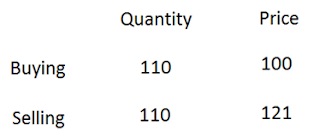➡ When the data is observed, we can see that 110 oranges are brought in Rs. 100 while 110 oranges are sold in Rs. 121. Ultimately, the person is having PROFIT in the entire transaction.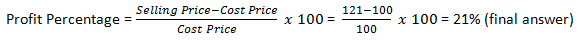➡ One more question will help you understand the process more clearly.

### Question 6

A man purchases 8 pens for Rs. 9 and sells 9 pens for Rs. 8. How much profit or loss does he make?
Solution: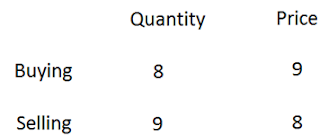➡ When we make the quantities equal,➡ When the data is observed, we can see that 72 oranges are bought in Rs. 81 while 72 oranges are sold in Rs. 64. Ultimately, the person is having LOSS in the entire transaction.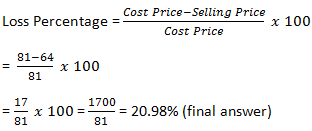### Few Practice problems:

1. Question: A boy buys oranges at 9 for Rs. 16 and sells them at 11 for Rs. 20. What is his gain or loss percentage?

Another type of question asked in examinations is a “dishonest dealer using false weight”. Let us try and understand this with an example.

### Question 7

A dishonest dealer professes to sell his goods at Cost Price, but he uses a weight of 960 gm for the kg weight. Find his gain percentage.
Solution:
➡ Since the kg weight is of 1000 gm but the dealer uses only the 960 gm weight. Thus giving himself the profit of
1000 – 960 = 40 gm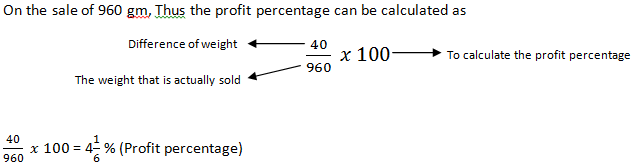### Few Practice Problems:

1. Question: A dishonest dealer professes to sell his goods at cost price, but he uses a weight of 950 gm for the kg weight. Find his gain percentage.
2. Question: A dishonest dealer professes to sell his goods at cost price but uses a weight of 875 gm for the kilogram weight. Determine his gain percentage.

### Question 8.

A dishonest dealer professes to sell his goods at a profit of 20% and also weighs 800 gm in place of a kg. Find his actual gain percentage?
Solution:
➡ Similar to our previous logic, he is suppose to weigh 1 kg i.e. 1000 gm instead he weighs 800 gm.So,
➡ Difference = 1000 – 800 = 200 gm
➡ Also he is selling his product at the profit of 20%. So we find 20% of 1000gm (1 kg).
20% of 1000 = 200 gm
➡ So, total profit = one from false weight + one from the profit percentage
➡ Profit = 200 + 200 = 400 gm
➡ What he actually sold = 800 gm.
➡ So, similar to the last question, the equation becomes,### Question 9

A merchant professes to sell his goods at a loss of 10% but weighs 750 gm in place of a kg. Find his real loss or gain percentage?
Solution:

➡ He is supposed to weigh 1000 gm instead he weighs 750 gm. So, profit equation be like
Difference = 1000 – 750 = 250
➡ But he is selling his goods at loss of 10%. So, we subtract this 10% of 1000 gm from the above profit.
➡ 10% of 1000 = 100 gm
➡ Total profit equation should look like,
➡ Total profit = 250 – 100 = 150 [we are saying profit here because the transaction results a positive value, which indicates the profit. If the transaction at the end results in a negative answer, the dealer will suffer a LOSS]
➡ On the sale of 750 gm. Therefore, similar to what we have been doing up untill now, the equation becomes➡ One another type of question is “To find the cost price”. Let’s have a look at the type of question and the way to solve them.

### Question 10

A man sells an article at 15% loss. If he had sold it for Rs. 450 more, he would have earned a profit of 10%. Find the cost price of this article.
Solution:

➡ Man when sells the article at loss,
➡ Loss percentage = x% = 15%
➡ Cost Price = 100
➡ Selling Price = 100 – x = 100 – 15 = 85
➡ Now, if he sells the article to earn the profit of 10%,
➡ Profit percentage = x% = 10%
➡ Cost Price = 100
➡ Selling Price = 100 + x = 100 + 10 = 110
➡ The difference in Selling price = 110 – 85 = 25
➡ And the sentence in the question says “if the product was sold for Rs. 450 more” which means the difference in amount is Rs. 450.
➡ Therefore, 25% = 450
➡ And we need to determine the Cost Price which is 100%, So
100% = ?
➡ The equation for calculation becomes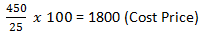➡ This method can be remembered in the form of formula as:### Few Practice Problems:

1. Question: A dealer sold a camera at a profit of 20%. Had he sold it for Rs. 200 less, he would have gained a profit of 15%. Determine the cost price.
2. Question: A man sells a bed enduring a loss of 80%. If he sells it for Rs. 72000 more, the loss percentage becomes 50%. Find the cost price of the bed.

In all the questions of above type, the COST PRICE of the article remained same while the condition was associated with SELLING PRICE. What if the condition mentioned in the question is associated with COST PRICE along with the SELLING PRICE. Let’s take a question to explore the type.

### Question 11

A person sells an article at a profit of 10%. If he had bought it at 10% less and sold it for Rs. 3 more, he would have gained 25%. Find the Cost Price?
Solution:
➡ Let us start solving this similar to what we have been doing up until now. We deal in percentages. ➡ Let us consider Cost Price of the article as 100%.
➡ First profit percentage = x% = 10%
➡ Cost Price = 100
➡ Selling Price = 100 + x = 100 + 10 = 110
➡ Next condition says that the Cost Price is reduced by 10%.
➡ 10% of intial Cost Price (100) = 10
➡ Cost Price = 100 – 10 = 90
➡ The line “he would have gained 25%” provides us with the profit percentage, when the cost price is 90.
➡ 25% of 90 = 22.5
➡ Since there is a profit.
➡ Cost Price + Profit = Selling Price
➡ 90 + 22.5 = 112.5
Let us make it visually easy for you to understand,
 First Condition Cost Price Selling Price 100 110 Second Condition Cost Price Selling Price 90 112.5

➡ Now, go through a condition in the question that says “sold it for Rs. 3 more”, this clearly indicates the difference in the two Selling Prices as in the Selling Price in first condition and the Selling Price in the second condition.
➡ Difference in the selling prices = Selling Price in first condition – Selling Price in second condition
➡ Difference = 112.5 – 110 = 2.5 [We just need the difference irrespective of sign]
➡ Now, according to our assumption, the difference is Rs. 2.5 but as per question, the difference must be Rs. 3. This is because of our assumption of COST PRICE initally. So,
➡ Cost Price (when the difference is 2.5) = 100
➡ Cost Price (when the difference is 3) =➡ So, the COST PRICE of the article is Rs. 120

Not to worry, go through the method one more time, you will get a hang of it.
Still let us solve one more similar type of problem to make the process crystal clear to you and then leave you with a practice problem to work on the process by yourself.

### Question 12

A man buys an article and sells it at a profit of 20%. If he had bought it at 20% less and sold it for Rs. 75 less, he would have gained 25%. What is the Cost Price of the article?
Solution:
➡ Let us start solving this similar to our process in last question. We deal in percentages. Let us consider Cost Price of the article as 100%.
➡ First profit percentage = x% = 20%
➡ Cost Price = 100
➡ Selling Price = 100 + x = 100 + 20 = 120
➡ Next condition says that the Cost Price is reduced by 20%.
➡ 20% of intial Cost Price (100) = 20
➡ Cost Price = 100 – 20 = 80
➡ The line “he would have gained 25%” provides us with the profit percentage, when the cost price is 80.
➡ 25% of 80 = 20
➡ Since, there is a profit.
➡ Cost Price + Profit = Selling Price
➡ 80 + 20 = 100
Let us make it visually easy for you to understand,
 First Condition Cost Price Selling Price 100 120 Second Condition Cost Price Selling Price 80 100

➡ Now, go through a condition in the question that says “sold it for Rs. 75 less”, this clearly indicates the difference in the two Selling Prices as in the Selling Price in first condition and the Selling Price in the second condition.

Difference in the selling prices = Selling Price in first condition – Selling Price in second condition
Difference = 120 – 100 = 20 [We just need the difference irrespective of sign]
➡ Now, according to our assumption, the difference is Rs. 20 but as per question, the difference must be Rs. 75. This is because of our assumption of COST PRICE initally. So,
Cost Price (when the difference is 20) = 100
➡ Cost Price (when the difference is 75) =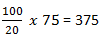➡ So, the COST PRICE of the article is Rs. 375

### Few Practice Problems:

1. Question: A person bought an article and sold it at a loss of 10%. If he had bought it for 20% less and sold it for Rs. 55 more, he would have had a profit of 40%. Find the Cost Price of the article? [Rs. 250]
2. Question: A man sells an article at a loss of 10%. If he had bought it at 20% less and sold it for Rs. 90 less, he would have made a profit of 10%. Find the Cost Price of the article? [Rs. 4500]

These are all the type of problems seen in the previous examinations and quicker methods to solve them. I really hope all of these are clear to you by now. Just traverse them again if necessary and solve all the problems that will help you solve all the different type of questions.
Before closing the chapter, I would like to provide you guys with some practice problems and hope you will solve them with utmost sincerity which will benefit you and you only.

### Point to Remember:

• Another term used in this topic is MARKED PRICE.
• Marked Price – This is the price which is marked by the salesperson to sell the product.
• Marked Price may or maynot be equal to Selling Price.
• Labelled Price is another name for Marked Price.
• If the term Marked Price is used in a question, make sure to take it in consideration.

### Question 13

Garima purchased a briefcase with an additional 10% discount on reduced price after deducting 20% on the Labelled Price. If the Labelled Price was Rs. 1400, then at what price did Garima purchase the briefcase?
Solution:
➡ In this too, let us work on the percentages to make our problems less troubling.
➡ Consider, Labelled Price = 100
➡ The salesperson had already announced the discount of 20% on the Labelled Price. So,
➡ 20% of Labelled Price (100) = 20
➡ Selling Price = 100 – 20 = 80
➡ But Garima got an additional 10% discount on the Selling Price which is 80 according to our calculation.
➡ 10% of Selling Price (80) = 8
➡ Selling Price for Garima = Selling Price – additional discount
➡ Selling Price for Garima = 80 – 8 = 72
➡ The Labelled Price is provided to us as Rs. 1400, which is 100% and we need to find the Selling ➡ Price for Garima, which is 72%. Therefore the equation should look like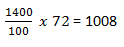➡ So, the Price at which Garima purchased the briefcase was Rs. 1008.

### Question 14

A tradesman marks his goods at 25% above his cost price and allows purchasers a discount offor cash. What profit does he make?### Question 15

A person sells two fans for Rs. 3200. The cost price of 1st fan is equal to the selling price of 2nd fan. If the 1st fan is sold at 40% loss and the second fan is sold at 100% gain. Find his overall profit or loss amount?
Solution:
➡ Total Selling Price of 2 fans = Rs. 3200
➡ Cost Price of 1st fan = Selling Price of 2nd fan
➡ Let us make a table to make it visually more clear,
 First fan Cost Price Selling Price 100 Second fan Cost Price Selling Price 100

➡ Now, the 1st fan is sold at 40% loss
➡ 40% of Cost Price (100) = 40
Therefore,
➡ Selling Price = 100 – 40 = 60
➡ As of 2nd fan, 100% is gained. Now, if the Selling Price of the fan is Rs. 100 after 100% profit, the Cost Price must have been
of 100 = 50
➡ Let us check it again.
➡ 100% is gained.
➡ Profit = 100% of Cost Price (50) = 50
➡ Sellling Price = Cost Price + Profit
➡ Selling Price = 50 + 50 = 100
➡ Let us now, fill out the table.
 First fan Cost Price Selling Price 100 60 Second fan Cost Price Selling Price 50 100

➡ Total Cost Price = 150
➡ Total Selling Price = 160
➡ Since, the total Selling Price is greater than the total Cost Price, the person must have had the
➡ Profit in the entire transaction. So,
➡ Profit = Total Selling Price – Total Cost Price
➡ Profit = 160 -150 = 10
➡ These are in percentages and we are provided with the Total Selling Price in amount, which is Rs. 3200. Which means
160% = 3200
10% = ?
➡ The final equation must look like,➡ This means the amount of profit the person had in the transaction is Rs. 200 (final answer).

Hope all this is clear to you. If you experience a problem in any of the questions given above, feel free to ask us in the comment section below. You can also share a problem from any other source. We will be more than happy to help you with it.

**BEST OF LUCK**